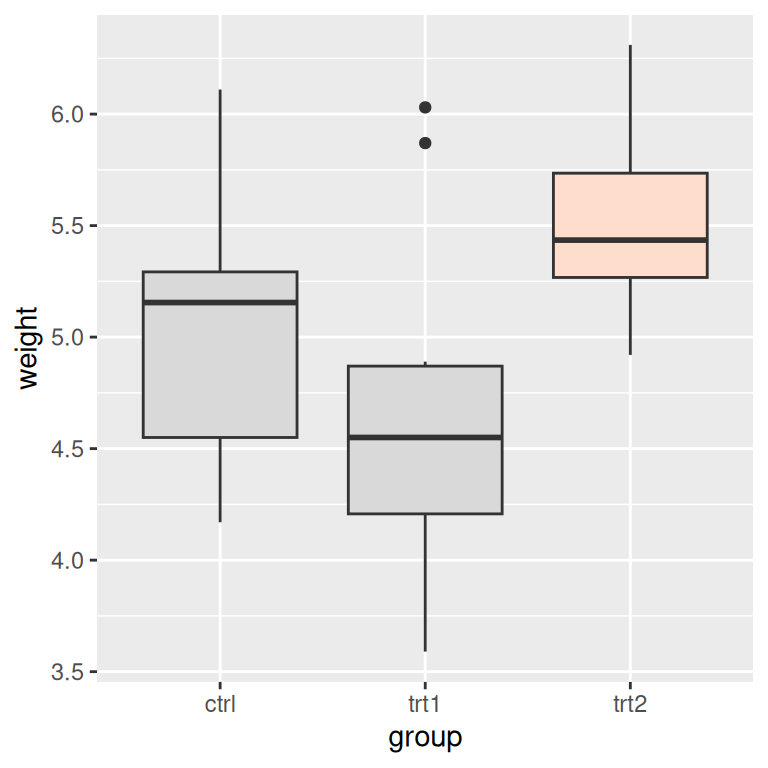## 7.6 Highlighting an Item

### 7.6.1 Problem

You want to change the color of an item to make it stand out.

### 7.6.2 Solution

To highlight one or more items, create a new column in the data and map it to the color. In this example, we’ll create a copy of the PlantGrowth data set called `pg_mod` and create a new column, `hl`, which is set to `no` if the case was in the control group or treatment 1 group, and set to `yes` if the case was in the treatment 2 group:

``````library(dplyr)

pg_mod <- PlantGrowth %>%
mutate(hl = recode(group, "ctrl" = "no", "trt1" = "no", "trt2" = "yes"))``````

Then we’ll plot this data with specified colors, and hiding the legend (Figure 7.13):

``````ggplot(pg_mod, aes(x = group, y = weight, fill = hl)) +
geom_boxplot() +
scale_fill_manual(values = c("grey85", "#FFDDCC"), guide = FALSE)``````Figure 7.13: Highlighting one item

### 7.6.3 Discussion

If you have a small number of items, as in this example, instead of creating a new column you could use the original one and specify the colors for every level of that variable. For example, the following code will use the group column from `PlantGrowth` and manually set the colors for each of the three levels. The result will appear the same as with the preceding code:

``````ggplot(PlantGrowth, aes(x = group, y = weight, fill = group)) +
geom_boxplot() +
scale_fill_manual(values = c("grey85", "grey85", "#FFDDCC"), guide = FALSE)``````